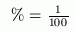﻿ Changing Decimals to Percents
CHANGING DECIMALS TO PERCENTS

One use for decimals is in working with percents, which are commonplace in everyday life:

• the dress was on sale for $\,40\%\,$ off the original price;
• housing costs rose $\,5\%\,$ last year;
• there was a $\,150\%\,$ increase in telephone activity after the newspaper advertisement.

There are $\,100\,$ cents in a dollar.
A century is $\,100\,$ years.
The word  percent  means  per one hundred .

The symbol  $\%$  is used for percent.
Whenever you see the symbol   $\%$ , you can trade it in for a factor of $\,\frac{1}{100}\,$.
Whenever you see a factor of $\,\frac{1}{100}\,$, it can be traded in for a  $\%$  symbol.
This simple idea is the key to success with percents:Indeed, the symbol  $\%$  even looks a bit like the fraction $\,\frac{1}{100}\,$;
it has the two zeros and the division bar!

To change from a decimal to a percent,
move the decimal point two places to the right and insert the percent symbol.

EXAMPLES:
$0.03 = 3\%$

$2.5 = 250\%$

$1.008 = 100.8\%$

Here's the idea that makes this work:

$\displaystyle 0.03 = \frac{3}{100} = 3\cdot\frac{1}{100} = 3\%$

Notice that the $\displaystyle\,\frac{1}{100}\,$ gets traded in for the percent symbol.

Master the ideas from this section
For example, $\,0.54\,$ gets renamed as $\,54\%\,$.